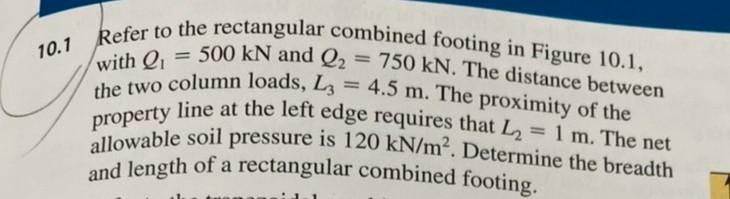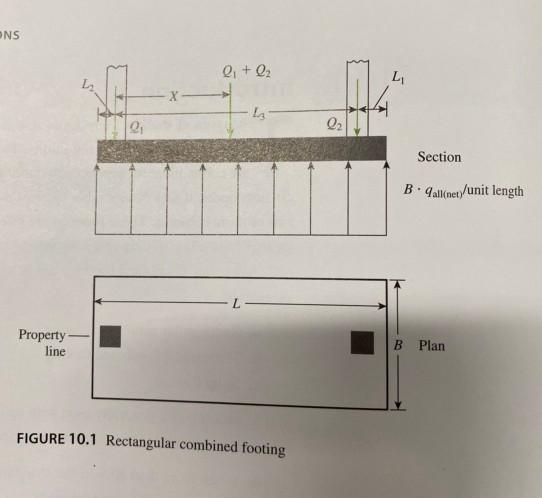# Question Refer to the rectangular combined footing in Figure 10.1, with = 500 kN and Q2 = 750 kN. The distance between the two column loads, L = 4.5 m. The proximity of the property line at the left edge requires that L2 = 1 m. The net allowable soil pressure is 120 kN/m². Determine the breadth and length of a rectangular combined footing. Q , La 10.1 ONS eit 0₂ L 02 Section Ballet/unit length L Property line B Plan FIGURE 10.1 Rectangular combined footingUJE4JE The Asker · Mechanical EngineeringTranscribed Image Text: Refer to the rectangular combined footing in Figure 10.1, with = 500 kN and Q2 = 750 kN. The distance between the two column loads, L = 4.5 m. The proximity of the property line at the left edge requires that L2 = 1 m. The net allowable soil pressure is 120 kN/m². Determine the breadth and length of a rectangular combined footing. Q , La 10.1 ONS eit 0₂ L 02 Section Ballet/unit length L Property line B Plan FIGURE 10.1 Rectangular combined footing
More
Transcribed Image Text: Refer to the rectangular combined footing in Figure 10.1, with = 500 kN and Q2 = 750 kN. The distance between the two column loads, L = 4.5 m. The proximity of the property line at the left edge requires that L2 = 1 m. The net allowable soil pressure is 120 kN/m². Determine the breadth and length of a rectangular combined footing. Q , La 10.1 ONS eit 0₂ L 02 Section Ballet/unit length L Property line B Plan FIGURE 10.1 Rectangular combined footing